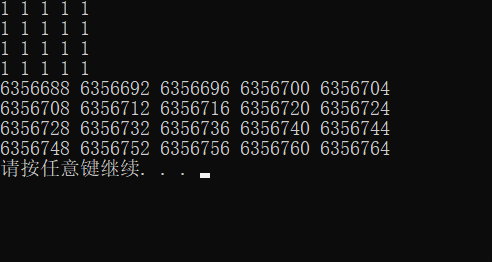2020-05-17 10:41

# C语言二维数组输出与输入值不符，想问下是哪里出错了？

``````#include<stdio.h>
int main()
{
int a;
int i,j;
for(i=0;i<=3;i++){
for(j=0;j<=4;j++){
scanf("%d",&a[i][j]);
}
}
for(i=0;i<=3;i++){
for(j=0;j<=4;j++){
printf("%d",&a[i][j]);
printf("\t");
}
printf("\n");
}
return 0;
}

``````• 点赞
• 写回答
• 关注问题
• 收藏
• 邀请回答